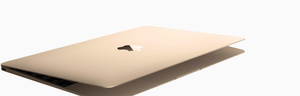## Refurbished Mac

Discover what goes into each refurbished Mac.A\$929.00

A\$1,189.00

A\$1,359.00

A\$1,609.00

A\$1,609.00

A\$1,679.00

A\$1,679.00

A\$1,739.00

A\$1,859.00

A\$1,859.00

A\$1,949.00

A\$1,999.00

A\$2,209.00

A\$2,209.00

A\$2,209.00

A\$2,359.00

A\$2,459.00

A\$2,549.00

A\$2,549.00

A\$2,679.00

A\$2,719.00

A\$2,799.00

A\$2,799.00

A\$2,799.00

A\$2,799.00

A\$2,919.00

A\$2,969.00

A\$2,969.00

A\$3,019.00

A\$3,059.00

A\$3,059.00

A\$3,059.00

A\$3,059.00

A\$3,059.00

A\$3,309.00

A\$3,309.00

A\$3,309.00

A\$3,369.00

A\$3,369.00

A\$3,369.00

A\$3,399.00

A\$3,569.00

A\$3,569.00

A\$3,569.00

A\$3,569.00

A\$3,639.00

A\$3,639.00

A\$3,779.00

A\$3,819.00

A\$3,869.00

A\$3,909.00

A\$3,929.00

A\$4,079.00

A\$4,079.00

A\$4,079.00

A\$4,179.00

A\$4,179.00

A\$4,329.00

A\$4,449.00

A\$4,589.00

A\$4,719.00

A\$4,719.00

A\$4,819.00

A\$4,839.00

A\$4,989.00

A\$5,079.00

A\$5,259.00

A\$5,259.00

A\$5,259.00

A\$5,529.00

A\$5,529.00

A\$5,589.00

A\$5,589.00

A\$5,799.00

A\$5,799.00

A\$6,069.00

A\$6,099.00

A\$6,339.00

A\$6,609.00

A\$6,609.00

A\$6,839.00

A\$7,689.00

A\$8,139.00

A\$8,229.00

A\$8,229.00

A\$8,389.00

A\$8,459.00